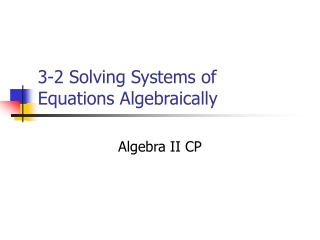DownloadDownload Presentation3-2 Solving Systems of Equations Algebraically

# 3-2 Solving Systems of Equations Algebraically

Download Presentation## 3-2 Solving Systems of Equations Algebraically

- - - - - - - - - - - - - - - - - - - - - - - - - - - E N D - - - - - - - - - - - - - - - - - - - - - - - - - - -
##### Presentation Transcript

1. 3-2 Solving Systems of Equations Algebraically Algebra II CP

2. Vocabulary • Substitution Method • Elimination Method

3. Ex 17: • Solve the system of equations by substitution x + 2y = 8 ½ x – y = 18

4. Ex : • Solve the system of equations by elimination 2x + y = 4 3x + y = 8

5. Ex : • Solve the system of equations by elimination 2x + 3y = 12 5x – 2y = 11

6. Ex last one: • Use either method to solve the system of equations 2x = 7 + y 6x – 3y = 24